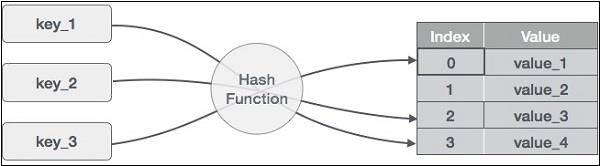# Hash Table Data Structure in Javascript

Web DevelopmentFront End TechnologyJavascript

Hash Table is a data structure which stores data in an associative manner. In a hash table, data is stored in an array format, where each data value has its own unique index value. Access to data becomes very fast if we know the index of the desired data.

Thus, it becomes a data structure in which insertion and search operations are very fast irrespective of the size of the data. Hash Table uses an array as a storage medium and uses the hash technique to generate an index where an element is to be inserted or is to be located from.

## Hashing

Hashing is a technique to convert a range of key values into a range of indexes of an array. We're going to use modulo operator to get a range of key values. Consider an example of a hash table of size 20, and the following items are to be stored. Item is in the (key, value) format.Here we have a hash function that takes in keys and generates indices for a table. These indices let us know where the value is stored. Now whenever we want to search for a value associated with a key, we just need to run the hash function on the key again and get the value in nearly constant time.

Hash functions are pretty hard to design though. Let's take an example −

Let's say we have the following hash function −

## Example

function modBy11(key) {
return key % 11;
}

And we start running this on key-value pairs we want to store, for example −

• (15, 20) - Hash code: 4
• (25, 39) - Hash code: 3
• (8, 55) - Hash code: 8
• (26, 84) - Hash code: 4

Here we see that we have a collision, ie, if we were to store 15 first and then encounter the key 26 with this hash function, it'll try to fix this entry in the same hole. This is called a collision. And to handle such situations, we need to define a collision handling mechanism. There are some well defined simple collision resolution algorithms. For example −

• Linear Probing: In this algorithm, we can search the next empty location in the array by looking into the next cell until we find an empty cell. In our example, since hole at 4 is taken, we can fill it in 5.
• Separate Chaining: In this implementation: We associate each location in the hash table with a list. Whenever we get a collision, we append the key-value pair at the end of this list. This can lead to much longer search times if chains keep getting longer.

Now that we understand how a hash table works and how we can use collision resolution, let's implement the HashTable class.

## Methods we'll implement

We'll implement these methods in our implementation −

• put(key, value): Adds a new key-value pair to the hash table
• get(key): Gets the value associated with a key
• remove(key): Removes the key-value pair from the table
• forEach(): Allows iterating over all key-value pairs
• static join(): A static method to join 2 hash tables in a new one